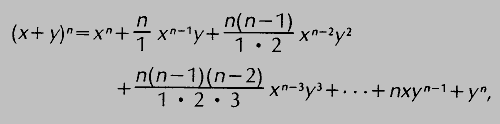# binomial

Also found in: Dictionary, Thesaurus, Medical, Financial, Wikipedia.

## binomial

(bī'nō`mēəl), polynomial expression (see polynomialpolynomial,
mathematical expression which is a finite sum, each term being a constant times a product of one or more variables raised to powers. With only one variable the general form of a polynomial is a0xn+a1x
) containing two terms, for example, x+y. The binomial theorem, or binomial formula, gives the expansion of the nth power of a binomial (x+y) for n=1, 2, 3, … , as follows:where the ellipsis (…) indicates a continuation of terms following the same pattern. For example, using the formula and reducing fractions, one obtains (x+y)5=x5+5x4y+10x3y2+10x2y3+5xy4+y5. The coefficients 1, n, n (n−1)/1·2, etc., of x and y may also be found from an array known as Pascal's triangle (for Blaise PascalPascal, Blaise
, 1623–62, French scientist and religious philosopher. Studying under the direction of his father, a civil servant, Pascal showed great precocity, especially in mathematics and science.
), formed by adding adjacent numbers to find the number below them as follows:## binomial

[bī′nō·mē·əl]
(mathematics)
A polynomial with only two terms.
McGraw-Hill Dictionary of Scientific & Technical Terms, 6E, Copyright © 2003 by The McGraw-Hill Companies, Inc.

## binomial

1. a mathematical expression consisting of two terms, such as 3x + 2y
2. a two-part taxonomic name for an animal or plant
3. referring to two names or terms
Collins Discovery Encyclopedia, 1st edition © HarperCollins Publishers 2005
References in periodicals archive ?
For comparison purposes, the Poisson and negative binomial models with log link function were fitted, their linear predictor was given by [[eta].sub.i] = [[beta].sub.0] + [[beta].sub.1][x.sub.i], and [x.sub.i] was the covariate described by age.
Based on the binomial tree completed by the forward process, the backward process calculates the option value.
Multiplying binomials and factoring related trinomials
Of the 3 data sets that used 4 classes and were not satisfactorily fit, one (Premeaux tier 2) approached statistical significance with the negative binomial distribution, but the other two (Premeaux tier 1 and McCabe) did not come close to a satisfactory fit.
Explanation of the steps to estimate PR using log-binomial regression models or Poisson regression models with robust variance in two statistical packages (STATA and R), using the tool bar or the specific syntax Package Tool Bar (step by step) Syntax unadjusted Log-Binomial regression Model STATA Menu tools[right arrow]Statistics[right arrow]Generalized Linear Models[right arrow]Generalized Lineal Models (GLM)[right arrow]Model [(dependent variable: auditc; independent variable: sex)/(family: binomial; link choices: log)] [right arrow]Reporting [report exponentiated coefficients.
The combined multinomial pricing model is obtained as follows: first, calculate the distribution series of the annualized volatility of the price of the mineral product through grouped statistical analysis; then, different values of the annualized volatility are calculated based on binomial pricing models; finally, these binomial models are combined into a multinomial pricing model based on the probability corresponding to each value of the annualized volatility values.
Previous studies suggested that using random parameters to mitigate the shortcomings of conventional models such as fixed Poisson and negative binomial models and to account for heterogeneity is beneficial even though the overall explanatory power of fixed and random parameter negative binomial models is similar [12, 14, 15].
This maxim in the title page of The Schoole, used as the motto of the book, encapsulates and announces some of the most important stylistic features of the text, including the use of the second person singular pronoun, of the imperative construction, and of syntactically and semantically parallel structures such as binomial phrases.
The k parameter of the negative binomial distribution estimated by the maximum likelihood method is calculated iteratively and is the value that equates the two members of the following (Bliss and Fisher, 1953):
Definition: A random variable X is said to follow Binomial distribution, if it assumes only nonnegative values and its probability mass function is given by,

Site: Follow: Share:
Open / Close